# Consider the following FSM state transition diagram: 7. Let's see if there is an equivalent state machine with fewe...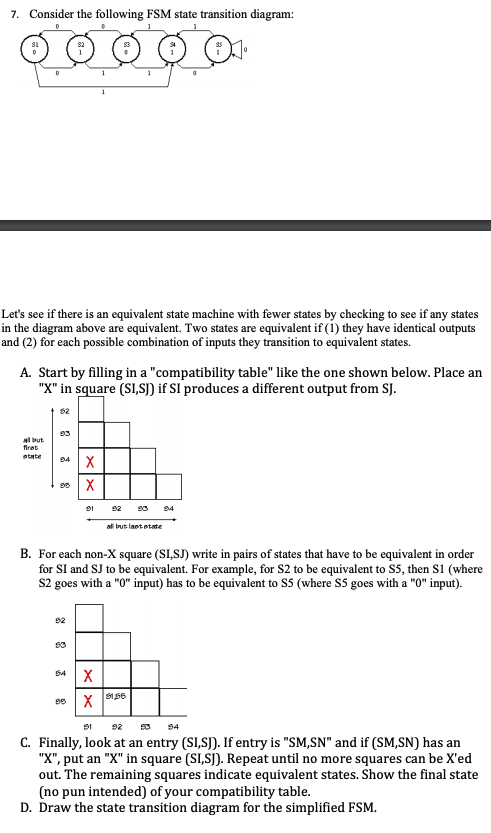Consider the following FSM state transition diagram: 7. Let's see if there is an equivalent state machine with fewer states by checking to see if any states in the diagram above are equivalent. Two states are equivalent if (1) they have identical outputs and (2) for each possible combination of inputs they transition to equivalent states. A. Start by filling in a "compatibility table" like the one shown below. Place an "X" in square (SISI) if SI produces a different output from SJ. 62 nl put 9 92 94 l but laetetate B. For each non-X square (SI,SJ) write in pairs of states that have to be equivalent in order for SI and SJ to be equivalent. For example, for S2 to be equivalent to SS, then S1 (where S2 goes with a "O" input) has to be equivalent to S5 (where S5 goes with a "O" input). 92 54 9ら C. Finally, look at an entry (SI,SI). If entry is "SM,SN" and if (SM,SN) has an "X", put an "X" in square (SLS). Repeat until no more squares can be X'ed out. The remaining squares indicate equivalent states. Show the final state (no pun intended) of your compatibility table. D. Draw the state transition diagram for the simplified FSM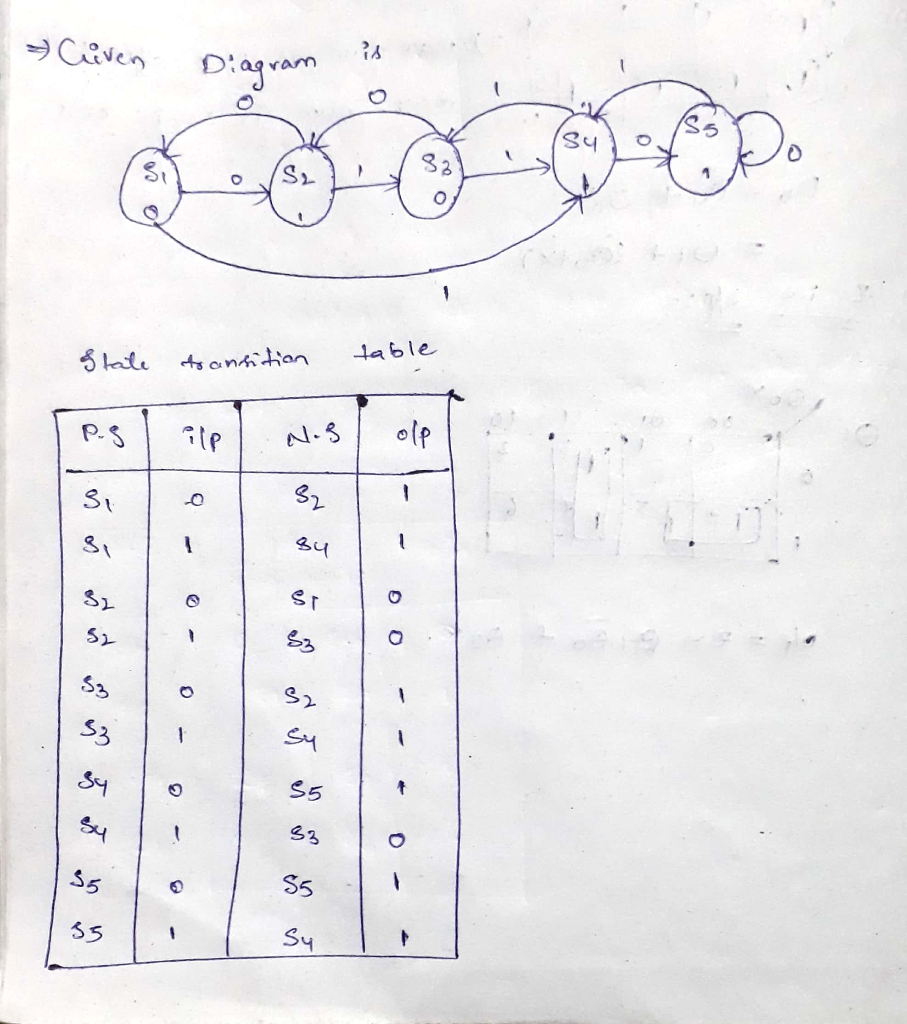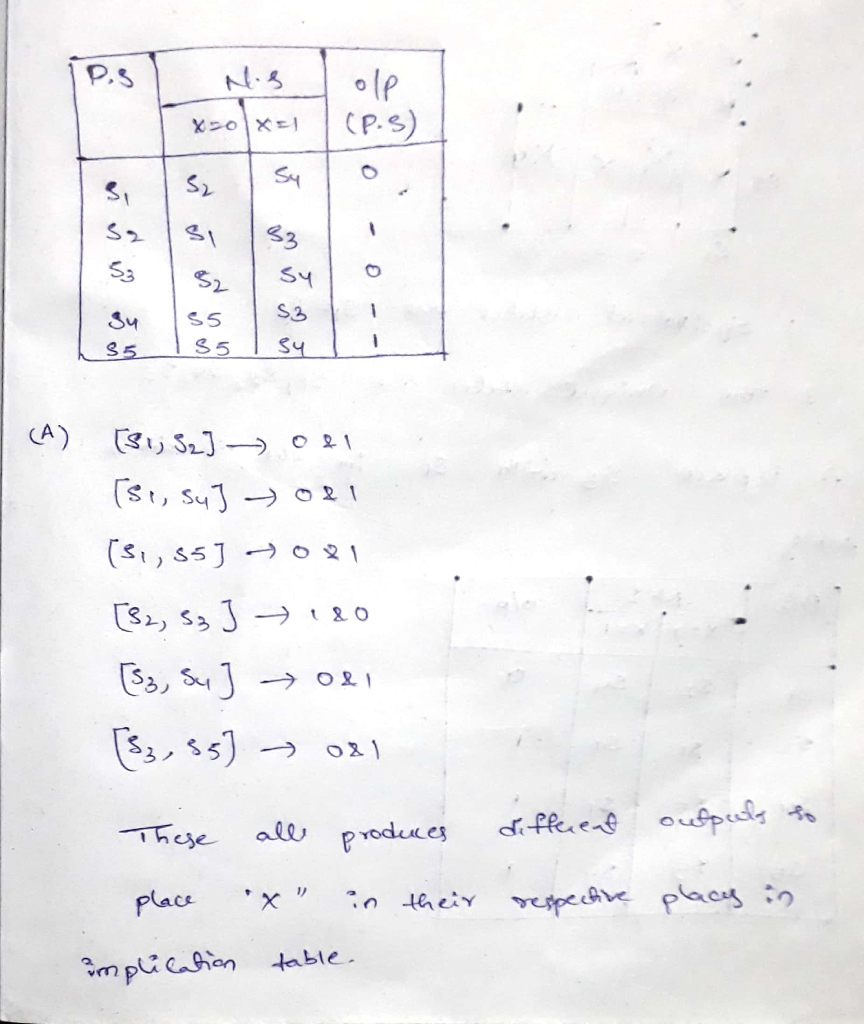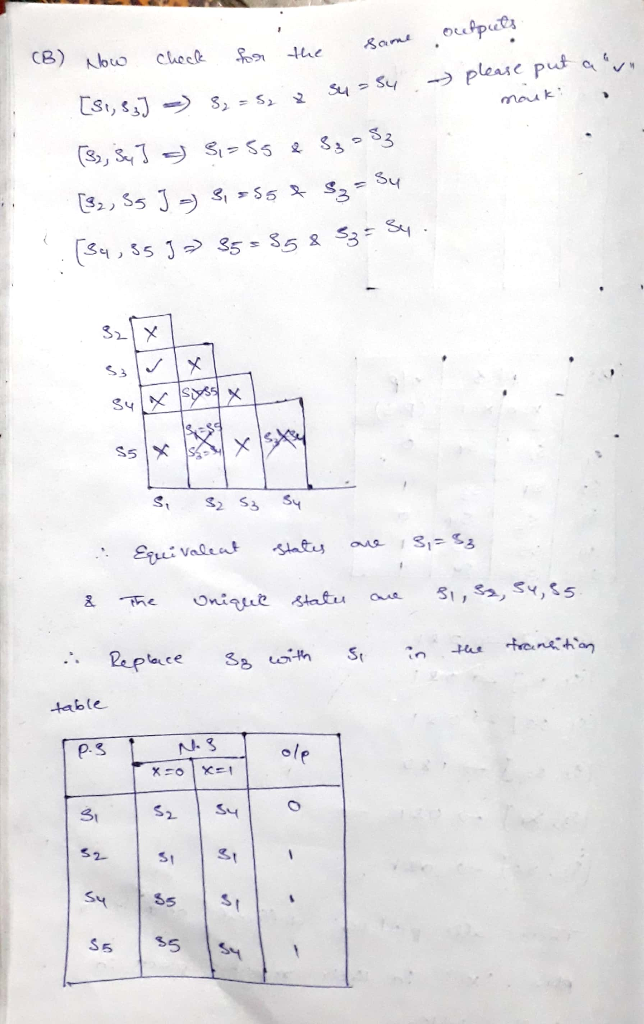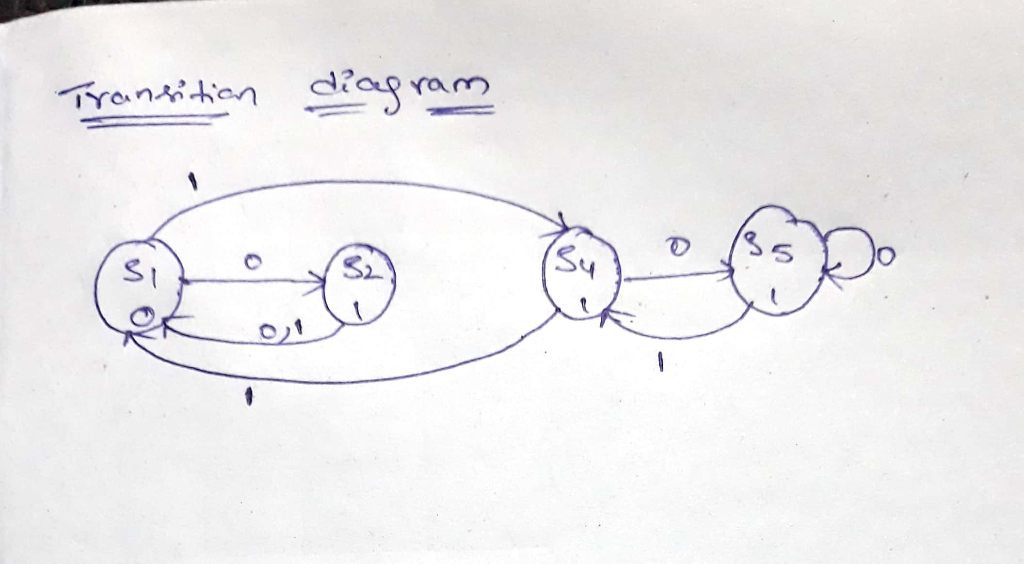##### Add Answer of: Consider the following FSM state transition diagram: 7. Let's see if there is an equivalent state machine with fewe...
More Homework Help Questions Additional questions in this topic.